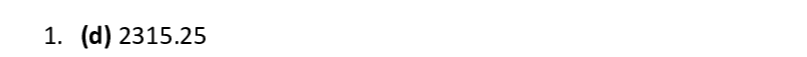Question

Financial Derivative And Risk Management

We invest 2000€ in a project which will generate an annual compound interest of 5%. What is the total amount that we will get after 3 years?

d. 2315,25

2300,25

c. 1315,25

None of the above is correct.Verified### Question 38679Financial Derivative And Risk Management

Which of the following bonds has the greatest price risk?
a. A 10-year \$100 annuity.
b. A 10-year, \$1,000 face value, zero coupon bond.
c. A 10-year, \$1,000 face value, 10% coupon bond with annual interest payments.
e. A 10-year, \$1,000 face value, 10% coupon bond with semiannual interest payments.
d. All 10-vear bonds have the same price risk since they have the same maturity,

### Question 38678Financial Derivative And Risk Management

A 10-year bond with a 9% annual coupon has a yield to maturity of 8%. Which of the following statements is CORRECT?
a. If the yield to maturity remains constant, the bond's price one year from now will be higher than its current price.
b. The bond is selling below its par value.
c. The bond is selling at a discount.
d. If the yield to maturity remains constant, the bond's price one year from now will be lower than its current price.
e. The bond's current yield is greater than 9%.

### Question 38677Financial Derivative And Risk Management

Which of the following statements is CORRECT?
a. If a coupon bond is selling at par, its current yield equals its yield to maturity.
b. If a coupon bond is selling at a discount, its price will continue to decline until it reaches its par value at maturity.
c. If interest rates increase, the price of a 10-year coupon bond will decline by a greater percentage than the price of a 10-year zero coupon bond.
d. If a bond's yield to maturity exceeds its annual coupon, then the bond will trade at a premium.
e. If a coupon bond is selling at a premium, its current yield equals its yield to maturity.

### Question 38676Financial Derivative And Risk Management

What are call provisions and sinking fund provisions? Do these provisions make bonds more or less risky?

### Question 38675Financial Derivative And Risk Management

Suppose Dillard Manufacturing sold an issue of bonds with a 10-year maturity, a \$1,000 face value, a 10% coupon rate, and semiannual interest payments.
a) Two years after the bonds were issued, the going rate of interest on bonds such as these fell to 6%. At what price would the bonds sell?
Suppose that further that the interest rate remained at 6% for the next 8 years. What would happen to the price of the bonds over time? Explain after the issue date (as in part a) interest rates fell to 6%. Suppose

### Question 38674Financial Derivative And Risk Management

The FAMA Company has two bond issues outstanding. Both bonds pay \$100 annual interestplus \$1000 face value at maturity. Bond L has a maturity of 15 years, and Bond S has a maturityof 1-year.
What will be the value of each of these bonds when the going rate of interest (yield to maturity)is:
A) 8%
B) 12%
Why does the longer-term (15-year) bond fluctuate more when interest rates change than does the shorter-term bond (1-year)?

### Question 38673Financial Derivative And Risk Management

Renfro Corporation's bonds will mature in 10 years. The bonds have a face value of \$1,000 and an 8 % coupon rate paid semiannually the price of the bonds is 1000 usd what is the bonds yield to maturity ,current yield and capital gains yield ?

### Question 38672Financial Derivative And Risk Management

GAMA Corp has issued bonds that have a 10 percent coupon rate payable semi annualy the bonds mature in 8 years have a face value of51000 and a yield to maturity of8.5 percent what is the price of the bonds

### Question 38575Financial Derivative And Risk Management

a) Explain the relationship between exchange rates, free capital movements and monetary policy autonomy, known as the "impossible/unholy trinity" or trilemma.(20 marks)
b) What does Rey (2015) mean when she argues that the trilemma is really a dilemma?

### Question 38574Financial Derivative And Risk Management

a) What are the main characteristics of money market instruments?
b) Who are traditionally expected to be the main issuers and holders of money market instruments? How has this changed in recent times? (5 marks)
c) The rate of discount is given as d = (R-P)/R.n
Where R is redemption value, P is initial price and n is time to redemption
If the current rate of discount on treasury bills is quoted at 3 per cent
Calculate the price of newly issued 13 week treasury bill, redemption value £500,000
If interest rates stay the same, what will happen to the price as the instrument approaches maturity? (5 marks)
What would happen to the price if interest rates rose? Demonstrate by calculating the price were interest rates 4% (5 marks)

### Submit query

Getting answers to your urgent problems is simple. Submit your query in the given box and get answers Instantly.

### Submit a new QuerySuccess

Assignment is successfully created